1596785930

# Have a Pacman Frog? Know-How to Take Care of Them

Pacman frogs are named after the famous Pacman game. The frogs have a figure like the Pacman monster. They are round, bright-colored, and have a wider mouth.

If you love frogs and toads and frogs, you can think of having a Pacman frog as your pet. They are quite entertaining to watch. In this article, you can get a comprehensive guide about how to give proper Pacman frog care.

Male Pacman frogs are smaller than females. They are a maximum of 4 inches in diameter. On the other hand, a female Pacman frog can reach up to 7inche in length and width. Additionally, they are long-living amphibians and can comfortably live for 15 years.
Pacman frogs are quite predatorial by nature. They can even attack and consume another Pacman frog or even a mate. So, it is better to keep them alone. But you can also keep a male and a female frog together.

** Their habitat**

Pacman frogs are initially from the dense and lush rainforest areas in Africa. So, they live in a humid and moist environment. A ten or a twenty-gallon aquarium is enough to keep a Pacman frog. You need to use a secure lid over the aquarium to prevent the frog from escaping.

They need a proper habitat. Otherwise, they may not live long and die even in a few months. You need to fill the base of the aquarium with a deep layer of the substrate. A moist substrate made with peat moss, coir, sphagnum moss, etc. is the best because it can retain moisture. You need to make it deep enough so that your pet frog can burrow inside it and rest there. So, for male frogs, 5 to six inches deep substrate is enough. For the female frogs, you need to use eight to ten inches substrate.

In addition to that, you need to recreate the atmosphere of the rainforest inside the aquarium. For those, you need to arrange the live plants densely. It will also provide your frog with a hiding place. Additionally, the live plants also help in retaining the moisture. The Pacman frogs absorb water through their skin. So, if the habitat is not humid enough, they might get dehydrated.

It is better to keep the terrarium away from direct sunlight. It can decrease the moisture level and will cause dehydration. So, keep the tank in a cool place away from direct sunlight.

You need to maintain the hygiene of their habitat. Or, tour frog might develop some illness. Make sure to clean the aquarium per week. If you are handling your pet, make sure to wash your hands and wear a glove. Oils of human skin are harmful to Pacman frogs. It also increases the risk of salmonella poisoning.

** Temperature and light**

Pacman frogs are ectotherms. That means they need an external source of heat to maintain their body temperature. In general, this species is comfortable in the ambient temperature ranging from 65 to 85 degrees F. You can use a broad-spectrum light bulb above the tank. It is excellent for APcman and other frogs. You need to provide 12 hours of light and dark per day so that your pet gets to maintain its biological clock.

**Food **

Pacman frogs are gluttons. They will eat anytime. But, you need to prevent overfeeding. Because Pacman frogs tend to overeat and will become obese. Pacman frogs eat insects, small mammals, snakes, reptiles, etc. You need to provide them with a variety of insects and other types of foods routinely. It will ensure your pet gets nutrition, protein, and vitamins in the proper dose,
You can provide small Pacman frogs crickets, mealworms, wax worms, small dubia roaches, etc. Adults and medium frogs eat pinkie mice, full-sized dubia roaches, etc. If you have an adult Pacman frog, provide them with small pice or pinkie rats. You need to deliver your frog’s food every day. Larger animals like pinkie mice, little mice, pinkie rats, etc. are excellent for once or twice per week.

Here is the essential guide for those who aim to have a Pacman frog as their pet. If you need more guidance, you can always visit a pet store or handler to get more information.

#pacman frog care

## Buddha Community1672928580

## How to Bash Read Command

Bash has no built-in function to take the user’s input from the terminal. The read command of Bash is used to take the user’s input from the terminal. This command has different options to take an input from the user in different ways. Multiple inputs can be taken using the single read command. Different ways of using this command in the Bash script are described in this tutorial.

## Syntax

The read command can be used without any argument or option. Many types of options can be used with this command to take the input of the particular data type. It can take more input from the user by defining the multiple variables with this command.

## Some Useful Options of the Read Command

Some options of the read command require an additional parameter to use. The most commonly used options of the read command are mentioned in the following:

## Different Examples of the Read Command

The uses of read command with different options are shown in this part of this tutorial.

Example 1: Using Read Command without Any Option and variable

Create a Bash file with the following script that takes the input from the terminal using the read command without any option and variable. If no variable is used with the read command, the input value is stored in the \$REPLY variable. The value of this variable is printed later after taking the input.

``````#!/bin/bash
#Print the prompt message
echo "Enter your favorite color: "
#Take the input
#Print the input value

Output:

The following output appears if the “Blue” value is taken as an input:Example 2: Using Read Command with a Variable

Create a Bash file with the following script that takes the input from the terminal using the read command with a variable. The method of taking the single or multiple variables using a read command is shown in this example. The values of all variables are printed later.

``````#!/bin/bash
#Print the prompt message
echo "Enter the product name: "
#Take the input with a single variable

#Print the prompt message
echo "Enter the color variations of the product: "
#Take three input values in three variables

#Print the input value
echo "The product name is \$item."
#Print the input values
echo "Available colors are \$color1, \$color2, and \$color3."``````

Output:

The following output appears after taking a single input first and three inputs later: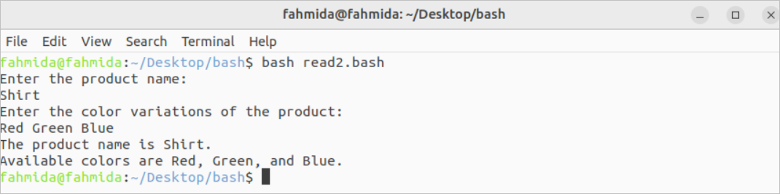Example 3: Using Read Command with -p Option

Create a Bash file with the following script that takes the input from the terminal using the read command with a variable and the -p option. The input value is printed later.

``````#!/bin/bash
#Take the input with the prompt message
read -p "Enter the book name: " book
#Print the input value
echo "Book name: \$book"``````

Output:

The following output appears after taking the input: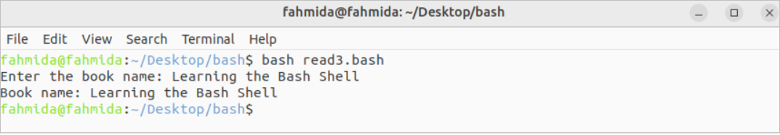Example 4: Using Read Command with -s Option

Create a Bash file with the following script that takes the input from the terminal using the read command with a variable and the -s option. The input value of the password will not be displayed for the -s option. The input values are checked later for authentication. A success or failure message is also printed.

``````#!/bin/bash
#Take the input with the prompt message
#Take the secret input with the prompt message

echo ""

#Check the email and password for authentication
then
#Print the success message
echo "Authenticated."
else
#Print the failure message
echo "Not authenticated."
fi``````

Output:

The following output appears after taking the valid and invalid input values: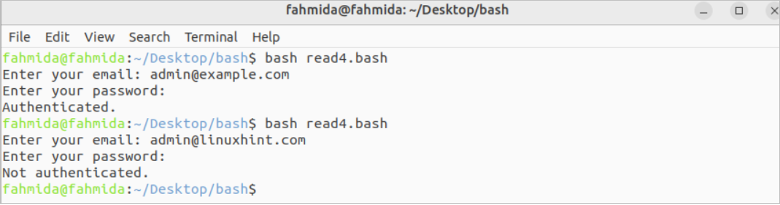Example 5: Using Read Command with -a Option

Create a Bash file with the following script that takes the input from the terminal using the read command with a variable and the -a option. The array values are printed later after taking the input values from the terminal.

``````#!/bin/bash
echo "Enter the country names: "
#Take multiple inputs using an array

echo "Country names are:"
for country in \${countries[@]}
do
echo \$country
done``````

Output:

The following output appears after taking the array values: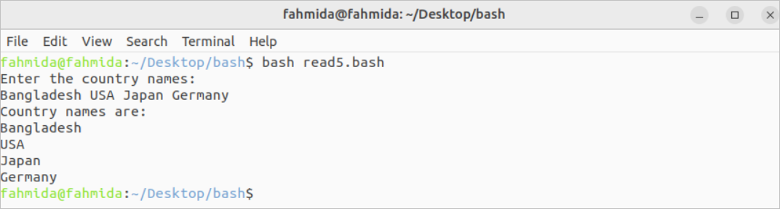Example 6: Using Read Command with -n Option

Create a Bash file with the following script that takes the input from the terminal using the read command with a variable and the -n option.

``````#!/bin/bash
#Print the prompt message
echo "Enter the product code: "
#Take the input of five characters
echo ""
#Print the input value
echo "The product code is \$code"``````

Output:

The following output appears if the “78342” value is taken as input: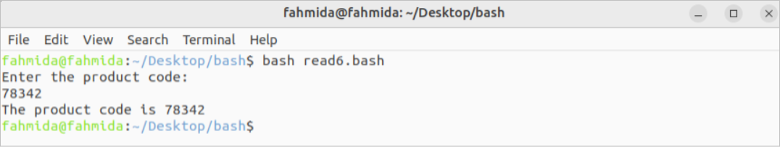Example 7: Using Read Command with -t Option

Create a Bash file with the following script that takes the input from the terminal using the read command with a variable and the -t option.

``````#!/bin/bash
#Print the prompt message
echo -n "Write the result of 10-6: "
#Take the input of five characters

#Check the input value
if [[ \$answer == "4" ]]
then
else
fi``````

Output:

The following output appears after taking the correct and incorrect input values: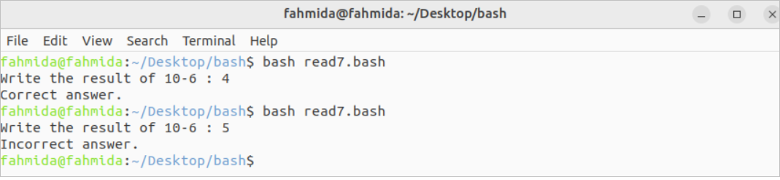## Conclusion

The uses of some useful options of the read command are explained in this tutorial using multiple examples to know the basic uses of the read command.

Original article source at: https://linuxhint.com/

1637595900

## Nesta postagem python, você aprenderá o seguinte:

• O que é uma pesquisa linear?
• Algoritmo de pesquisa linear
• Escreva um programa Python para pesquisa linear usando loop while
• Escreva um programa Python para pesquisa linear usando o loop for
• Pesquisa linear no programa Python usando recursão

### O que é uma pesquisa linear?

Em primeiro lugar, uma pesquisa linear, também conhecida como pesquisa sequencial, este método é usado para localizar um elemento dentro de uma lista ou array. Ele verifica cada elemento da lista um por um / sequencialmente até que uma correspondência seja encontrada ou toda a lista tenha sido pesquisada.

### Algoritmo de pesquisa linear

Implemente a pesquisa linear seguindo as etapas abaixo:

• Percorra a lista / array usando um loop.
• Em cada iteração, associe o `target` valor ao  valor fornecido da lista / matriz.
• Se os valores corresponderem, retorne o índice atual da lista / matriz.
• Caso contrário, vá para o próximo elemento de array / lista.
• Se nenhuma correspondência for encontrada, retorne  `-1`.

### Escreva um programa Python para pesquisa linear usando loop while

``````# python program for linear search using while loop

#define list
lst = []

#take input list size
num = int(input("Enter size of list :- "))

for n in range(num):
#append element in list/array
numbers = int(input("Enter the array of %d element :- " %n))
lst.append(numbers)

#take input number to be find in list
x = int(input("Enter number to search in list :- "))

i = 0
flag = False

while i < len(lst):
if lst[i] == x:
flag = True
break

i = i + 1

if flag == 1:
print('{} was found at index {}.'.format(x, i))
else:
``````

Depois de executar o programa, a saída será:

``````Enter size of list :-  5
Enter the array of 0 element :-  10
Enter the array of 1 element :-  23
Enter the array of 2 element :-  56
Enter the array of 3 element :-  89
Enter the array of 4 element :-  200
Enter number to search in list :-  89
89 was found at index 3.
``````

### Escreva um programa Python para pesquisa linear usando o loop for

``````# python program for linear search using for loop

#define list
lst = []

#take input list size
num = int(input("Enter size of list :- "))

for n in range(num):
#append element in list/array
numbers = int(input("Enter the array of %d element :- " %n))
lst.append(numbers)

#take input number to be find in list
x = int(input("Enter number to search in list :- "))

i = 0
flag = False

for i in range(len(lst)):
if lst[i] == x:
flag = True
break

if flag == 1:
print('{} was found at index {}.'.format(x, i))
else:

``````

Depois de executar o programa, a saída será:

``````Enter size of list :-  6
Enter the array of 0 element :-  25
Enter the array of 1 element :-  50
Enter the array of 2 element :-  100
Enter the array of 3 element :-  200
Enter the array of 4 element :-  250
Enter the array of 5 element :-  650
Enter number to search in list :-  200
200 was found at index 3.

``````

### Pesquisa linear no programa Python usando recursão

``````# python program for linear search using for loop

#define list
lst = []

#take input list size
num = int(input("Enter size of list :- "))

for n in range(num):
#append element in list/array
numbers = int(input("Enter the array of %d element :- " %n))
lst.append(numbers)

#take input number to be find in list
x = int(input("Enter number to search in list :- "))

# Recursive function to linear search x in arr[l..r]
def recLinearSearch( arr, l, r, x):
if r < l:
return -1
if arr[l] == x:
return l
if arr[r] == x:
return r
return recLinearSearch(arr, l+1, r-1, x)

res = recLinearSearch(lst, 0, len(lst)-1, x)

if res != -1:
print('{} was found at index {}.'.format(x, res))
else:

``````

Depois de executar o programa, a saída será:

``````Enter size of list :-  5
Enter the array of 0 element :-  14
Enter the array of 1 element :-  25
Enter the array of 2 element :-  63
Enter the array of 3 element :-  42
Enter the array of 4 element :-  78
Enter number to search in list :-  78
78 was found at index 4.``````1637563159

## Trong bài đăng python này, bạn sẽ tìm hiểu những điều sau:

• Tìm kiếm tuyến tính là gì?
• Thuật toán tìm kiếm tuyến tính
• Viết chương trình Python để tìm kiếm tuyến tính bằng While Loop
• Viết chương trình Python để tìm kiếm tuyến tính bằng For Loop
• Tìm kiếm tuyến tính trong Chương trình Python sử dụng Đệ quy

### Tìm kiếm tuyến tính là gì?

Trước hết, Tìm kiếm tuyến tính, còn được gọi là tìm kiếm tuần tự, phương pháp này được sử dụng để tìm một phần tử trong danh sách hoặc mảng. Nó kiểm tra từng phần tử của danh sách một / tuần tự cho đến khi tìm thấy một kết quả phù hợp hoặc toàn bộ danh sách đã được tìm kiếm.

### Thuật toán tìm kiếm tuyến tính

Triển khai tìm kiếm tuyến tính theo các bước sau:

• Duyệt qua danh sách / mảng bằng cách sử dụng một vòng lặp.
• Trong mỗi lần lặp, hãy liên kết  `target` giá trị với giá trị đã cho của danh sách / mảng.
• Nếu các giá trị khớp, trả về chỉ mục hiện tại của danh sách / mảng.
• Nếu không, hãy chuyển sang phần tử mảng / danh sách tiếp theo.
• Nếu không tìm thấy kết quả phù hợp, hãy quay lại  `-1`.

### Viết chương trình Python để tìm kiếm tuyến tính bằng While Loop

``````# python program for linear search using while loop

#define list
lst = []

#take input list size
num = int(input("Enter size of list :- "))

for n in range(num):
#append element in list/array
numbers = int(input("Enter the array of %d element :- " %n))
lst.append(numbers)

#take input number to be find in list
x = int(input("Enter number to search in list :- "))

i = 0
flag = False

while i < len(lst):
if lst[i] == x:
flag = True
break

i = i + 1

if flag == 1:
print('{} was found at index {}.'.format(x, i))
else:
``````

Sau khi thực hiện chương trình, kết quả đầu ra sẽ là:

``````Enter size of list :-  5
Enter the array of 0 element :-  10
Enter the array of 1 element :-  23
Enter the array of 2 element :-  56
Enter the array of 3 element :-  89
Enter the array of 4 element :-  200
Enter number to search in list :-  89
89 was found at index 3.
``````

### Viết chương trình Python để tìm kiếm tuyến tính bằng For Loop

``````# python program for linear search using for loop

#define list
lst = []

#take input list size
num = int(input("Enter size of list :- "))

for n in range(num):
#append element in list/array
numbers = int(input("Enter the array of %d element :- " %n))
lst.append(numbers)

#take input number to be find in list
x = int(input("Enter number to search in list :- "))

i = 0
flag = False

for i in range(len(lst)):
if lst[i] == x:
flag = True
break

if flag == 1:
print('{} was found at index {}.'.format(x, i))
else:

``````

Sau khi thực hiện chương trình, kết quả đầu ra sẽ là:

``````Enter size of list :-  6
Enter the array of 0 element :-  25
Enter the array of 1 element :-  50
Enter the array of 2 element :-  100
Enter the array of 3 element :-  200
Enter the array of 4 element :-  250
Enter the array of 5 element :-  650
Enter number to search in list :-  200
200 was found at index 3.

``````

### Tìm kiếm tuyến tính trong Chương trình Python sử dụng Đệ quy

``````# python program for linear search using for loop

#define list
lst = []

#take input list size
num = int(input("Enter size of list :- "))

for n in range(num):
#append element in list/array
numbers = int(input("Enter the array of %d element :- " %n))
lst.append(numbers)

#take input number to be find in list
x = int(input("Enter number to search in list :- "))

# Recursive function to linear search x in arr[l..r]
def recLinearSearch( arr, l, r, x):
if r < l:
return -1
if arr[l] == x:
return l
if arr[r] == x:
return r
return recLinearSearch(arr, l+1, r-1, x)

res = recLinearSearch(lst, 0, len(lst)-1, x)

if res != -1:
print('{} was found at index {}.'.format(x, res))
else:

``````

Sau khi thực hiện chương trình, kết quả đầu ra sẽ là:

``````Enter size of list :-  5
Enter the array of 0 element :-  14
Enter the array of 1 element :-  25
Enter the array of 2 element :-  63
Enter the array of 3 element :-  42
Enter the array of 4 element :-  78
Enter number to search in list :-  78
78 was found at index 4.``````

1637592240

## In diesem Python-Beitrag erfahren Sie Folgendes:

• Was ist eine lineare Suche?
• Linearer Suchalgorithmus
• Schreiben Sie ein Python-Programm für die lineare Suche mit While-Schleife
• Schreiben Sie ein Python-Programm für die lineare Suche mit der For-Schleife
• Lineare Suche im Python-Programm mit Rekursion

### Was ist eine lineare Suche?

Eine lineare Suche, auch bekannt als sequentielle Suche, diese Methode wird verwendet, um ein Element innerhalb einer Liste oder eines Arrays zu finden. Es überprüft jedes Element der Liste nacheinander / sequentiell, bis eine Übereinstimmung gefunden wird oder die gesamte Liste durchsucht wurde.

### Linearer Suchalgorithmus

Implementieren Sie die lineare Suche mit den folgenden Schritten:

• Durchlaufen Sie die Liste/das Array mit einer Schleife.
• Verknüpfen Sie in jeder Iteration den  `target` Wert mit dem angegebenen Wert der Liste/des Arrays.
• Wenn die Werte übereinstimmen, geben Sie den aktuellen Index der Liste/des Arrays zurück.
• Fahren Sie andernfalls mit dem nächsten Array-/Listenelement fort.
• Wenn keine Übereinstimmung gefunden wird, geben Sie zurück  `-1`.

### Schreiben Sie ein Python-Programm für die lineare Suche mit While-Schleife

``````# python program for linear search using while loop

#define list
lst = []

#take input list size
num = int(input("Enter size of list :- "))

for n in range(num):
#append element in list/array
numbers = int(input("Enter the array of %d element :- " %n))
lst.append(numbers)

#take input number to be find in list
x = int(input("Enter number to search in list :- "))

i = 0
flag = False

while i < len(lst):
if lst[i] == x:
flag = True
break

i = i + 1

if flag == 1:
print('{} was found at index {}.'.format(x, i))
else:
``````

Nach der Ausführung des Programms lautet die Ausgabe:

``````Enter size of list :-  5
Enter the array of 0 element :-  10
Enter the array of 1 element :-  23
Enter the array of 2 element :-  56
Enter the array of 3 element :-  89
Enter the array of 4 element :-  200
Enter number to search in list :-  89
89 was found at index 3.
``````

### Schreiben Sie ein Python-Programm für die lineare Suche mit der For-Schleife

``````# python program for linear search using for loop

#define list
lst = []

#take input list size
num = int(input("Enter size of list :- "))

for n in range(num):
#append element in list/array
numbers = int(input("Enter the array of %d element :- " %n))
lst.append(numbers)

#take input number to be find in list
x = int(input("Enter number to search in list :- "))

i = 0
flag = False

for i in range(len(lst)):
if lst[i] == x:
flag = True
break

if flag == 1:
print('{} was found at index {}.'.format(x, i))
else:

``````

Nach der Ausführung des Programms lautet die Ausgabe:

``````Enter size of list :-  6
Enter the array of 0 element :-  25
Enter the array of 1 element :-  50
Enter the array of 2 element :-  100
Enter the array of 3 element :-  200
Enter the array of 4 element :-  250
Enter the array of 5 element :-  650
Enter number to search in list :-  200
200 was found at index 3.

``````

### Lineare Suche im Python-Programm mit Rekursion

``````# python program for linear search using for loop

#define list
lst = []

#take input list size
num = int(input("Enter size of list :- "))

for n in range(num):
#append element in list/array
numbers = int(input("Enter the array of %d element :- " %n))
lst.append(numbers)

#take input number to be find in list
x = int(input("Enter number to search in list :- "))

# Recursive function to linear search x in arr[l..r]
def recLinearSearch( arr, l, r, x):
if r < l:
return -1
if arr[l] == x:
return l
if arr[r] == x:
return r
return recLinearSearch(arr, l+1, r-1, x)

res = recLinearSearch(lst, 0, len(lst)-1, x)

if res != -1:
print('{} was found at index {}.'.format(x, res))
else:

``````

Nach der Ausführung des Programms lautet die Ausgabe:

``````Enter size of list :-  5
Enter the array of 0 element :-  14
Enter the array of 1 element :-  25
Enter the array of 2 element :-  63
Enter the array of 3 element :-  42
Enter the array of 4 element :-  78
Enter number to search in list :-  78
78 was found at index 4.``````1636296420

## 線形検索のためのPythonプログラム

このチュートリアルでは、Pythonで線形検索プログラムを作成する方法を学習します。

まず、線形検索（シーケンシャル検索とも呼ばれます）は、リストまたは配列内の要素を見つけるために使用されます。一致するものが見つかるか、リスト全体が検索されるまで、リストの各要素を1つずつ/順番にチェックします。

### 線形探索アルゴリズム

• ループを使用してリスト/配列をトラバースします。
• すべての反復で、`target` 値をリスト/配列の指定された値に関連付け ます。
• 値が一致する場合は、リスト/配列の現在のインデックスを返します。
• それ以外の場合は、次の配列/リスト要素に移動します。
• 一致するものが見つからない場合は、を返し `-1`ます。

## 線形検索のためのPythonプログラム

• whileループを使用した線形検索用のPythonプログラム
• Forループを使用した線形検索用のPythonプログラム
• 再帰を使用したPythonプログラムでの線形検索

### whileループを使用した線形検索用のPythonプログラム

``````# python program for linear search using while loop

#define list
lst = []

#take input list size
num = int(input("Enter size of list :- "))

for n in range(num):
#append element in list/array
numbers = int(input("Enter the array of %d element :- " %n))
lst.append(numbers)

#take input number to be find in list
x = int(input("Enter number to search in list :- "))

i = 0
flag = False

while i < len(lst):
if lst[i] == x:
flag = True
break

i = i + 1

if flag == 1:
print('{} was found at index {}.'.format(x, i))
else:
``````

プログラムの実行後、出力は次のようになります。

``````Enter size of list :-  5
Enter the array of 0 element :-  10
Enter the array of 1 element :-  23
Enter the array of 2 element :-  56
Enter the array of 3 element :-  89
Enter the array of 4 element :-  200
Enter number to search in list :-  89
89 was found at index 3.``````

### Forループを使用した線形検索用のPythonプログラム

``````# python program for linear search using for loop

#define list
lst = []

#take input list size
num = int(input("Enter size of list :- "))

for n in range(num):
#append element in list/array
numbers = int(input("Enter the array of %d element :- " %n))
lst.append(numbers)

#take input number to be find in list
x = int(input("Enter number to search in list :- "))

i = 0
flag = False

for i in range(len(lst)):
if lst[i] == x:
flag = True
break

if flag == 1:
print('{} was found at index {}.'.format(x, i))
else:
``````

プログラムの実行後、出力は次のようになります。

``````Enter size of list :-  6
Enter the array of 0 element :-  25
Enter the array of 1 element :-  50
Enter the array of 2 element :-  100
Enter the array of 3 element :-  200
Enter the array of 4 element :-  250
Enter the array of 5 element :-  650
Enter number to search in list :-  200
200 was found at index 3.``````

### 再帰を使用したPythonプログラムでの線形検索

``````# python program for linear search using for loop

#define list
lst = []

#take input list size
num = int(input("Enter size of list :- "))

for n in range(num):
#append element in list/array
numbers = int(input("Enter the array of %d element :- " %n))
lst.append(numbers)

#take input number to be find in list
x = int(input("Enter number to search in list :- "))

# Recursive function to linear search x in arr[l..r]
def recLinearSearch( arr, l, r, x):
if r < l:
return -1
if arr[l] == x:
return l
if arr[r] == x:
return r
return recLinearSearch(arr, l+1, r-1, x)

res = recLinearSearch(lst, 0, len(lst)-1, x)

if res != -1:
print('{} was found at index {}.'.format(x, res))
else:
``````Enter size of list :-  5